# Class 12 Maths NCERT Solutions for Chapter 13 Probability Exercise 13.2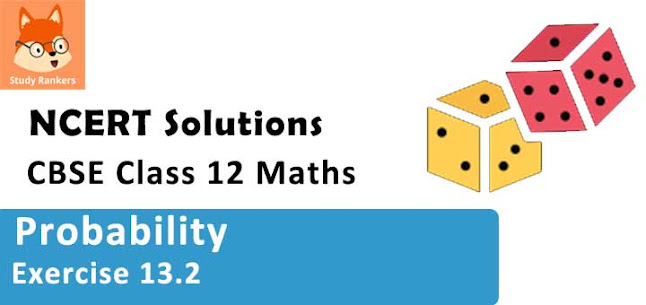### Probability Exercise 13.2 Solutions

1. If P(A) = 3/5 and P(B) = 1/5, find P(A ∩ B) if A and B are independent events.

Solution

It is given that P(A) = 3/5 and P(B) = 1/5
A and B are independent events. Therefore,
P(A ∩ B) = P(A) . P(B) = 3/5. 1/5 = 3/25

2. Two cards are drawn at random and without replacement from a pack of 52 playing cards. Find the probability that both the cards are black.

Solution

There are 26 black cards in a deck of 52 cards.
Let P (A) be the probability of getting a black card in the first draw.
∴ P(A) = 26/52 = 1/2
Let P(B) be the probability of getting a black card on the second draw.
Since the card is not replaced,
∴ P(B) = 25/51
Thus, probability of getting both the cards black = (1/2) × (25/51) = 25/102

3. A box of oranges is inspected by examining three randomly selected oranges drawn without replacement. If all the three oranges are good, the box is approved for sale, otherwise, it is rejected. Find the probability that a box containing 15 oranges out of which 12 are good and 3 are bad ones will be approved for sale

Solution

Let A, B, and C be the respective events that the first, second, and third drawn orange is good.
Therefore, probability that first drawn orange is good, P (A) = 12/15
The oranges are not replaced.
Therefore, probability of getting second orange good, P (B) = 11/14
Similarly, probability of getting third orange good, P(C) = 10/13
The box is approved for sale, if all the three oranges are good.
Thus, probability of getting all the oranges good = 12/15 × 11/14 × 10/13 = 44/91
Therefore, the probability that the box is approved for sale is = 44/91.

4. A fair coin and an unbiased die are tossed. Let A be the event ‘head appears on the coin’ and B be the event ‘3 on the die’. Check whether A and B are independent events or not.

Solution

If a fair coin and an unbiased die are tossed, then the sample space S is given by,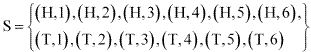Let A: Head appears on the coin
A = {(H,1), (H, 2), (H, 3), (H, 4), (H, 5), (H, 6)}
⇒ P(A) = 6/12 = 1/2
B :  3 on die = { (H, 3), (T, 3)}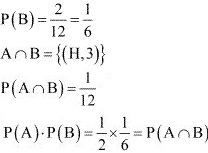Therefore, A and B are independent events.

5. A die, whose faces are marked 1, 2, 3 in red and 4, 5, 6 in green is tossed. Let A be the event "number obtained is even" and B be the event "number obtained is red". Find if A and B are independent events.

Solution

When a die is thrown, the sample space (S) is
S = {1, 2, 3, 4, 5, 6}
Let A: the number is even = {2, 4, 6}
⇒ P(A) = 3/6 = 1/2
B : the number is red = {1, 2, 3}
⇒ PB =36=12∴ A ∩ B = {2}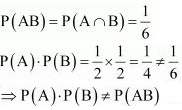Therefore, A and B are not independent.

6. Let E and F be events with P(E) = 3/5, P(F) = 3/10 and P(E∩ F ) = 1/5. Are E and F independent ?

Solution

It is given that P(E) = 3/5, P(F) = 3/10 and P(EF) = P(E∩ F) = 1/5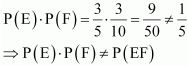Therefore, E and F are not independent.

7. Given that the events A and B are such that P(A) = 12, PA∪B=35 and P (B) = p. Find p if they are (i) mutually exclusive (ii) independent.

Solution

It is given that P(A) = 1/2, P(A∪B) = 3/5, and P(B) = p
(i) When A and B are mutually exclusive, A∩B = Ø
P(A∩B) = 0
It is known that, P(A∪B) = P(A) + P(B) - P(A∩B)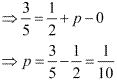(ii) When A and B are independent, P(A∩B) = P(A). P(B) = (1/2)P
It is known that, P(A∪B) = P(A) + P(B)  - P(A∩B)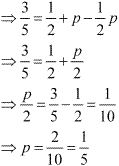8. Let A and B be independent events with P (A) = 0.3 and P (B) = 0.4. Find
(i) P (A ∩ B)
(ii) P (A ∪ B)
(iii) P (A | B)
(iv) P (B | A)

Solution

It is given that P (A) = 0.3 and P (B) = 0.4
(i) If A and B are independent events, then
P(A ∩ B) = P(A).P(B) = 0.3 × 0.4 = 0.12

(ii) It is known that, P(A ∪ B) = P(A) + P(B) - P(A ∩ B)
⇒ P(A ∪ B) = 0.3 + 0.4 - 0.12 = 0.58

(iii) It is known that, P(A|B) = P(A ∩ B)/P(B)
⇒ P(A|B) = 0.12/0.4 = 0.3

(iv) It is known that, P(B|A) = P(A ∩ B)/P(A)
⇒ P(B|A) = 0.12/0.3 = 0.4

9. If A and B are two events such that P(A) = 1/4, P(B) = 1/2 and and P(A ∩ B) = 1/8, find P(not A and not B)

Solution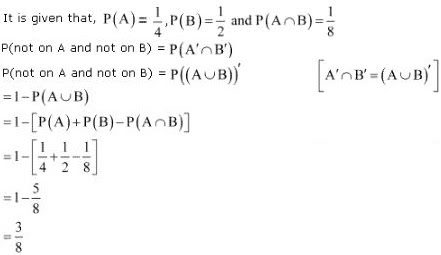10. Events A and B are such that P(A) = 1/2, P(B) = 7/12 and P(not A or not B) = 1/4 . State whether A and B are independent ?

Solution

It is given that P(A) = 1/2, P(B) = 7/12, and P(not A or not B) = 1/4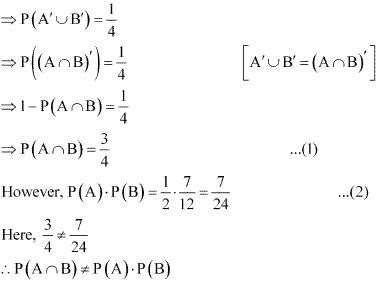Therefore, A and B are independent events.

11. Given two independent events A and B such that P (A) = 0.3, P (B) = 0.6. Find
(i) P (A and B)
(ii) P (A and not B)
(iii) P (A or B)
(iv) P (neither A nor B)

Solution

It is given that P(A) = 0.3 and P(B) = 0.6
Also, A and B are independent events,
(i) ∴ P(A and B) = P(A). P(B)
⇒ P(A  ∩ B) = 0.3 × 0.6 = 0.18

(ii) P(A and not B) = P(A ∩ B')
= P(A) - P(A ∩ B)
= 0.3 - 0.18
= 0.12

(iii) P( A or B) = P(A ∪ B)
= P(A) + P(B)  - P(A∩B)
= 0.3 + 0.6 - 0.18
= 0.72

(iv) P (neither A nor B) = P(A' ∩ B')
= P[(A ∪ B)']
= 1 - P(A ∪ B)
= 1 - 0.72
= 0.28

12. A die is tossed thrice. Find the probability of getting an odd number at least once.

Solution

Probability of getting an odd number in a single throw of a die = 3/6 = 1/2
Similarly, probability of getting an even number = 3/6 = 1/2
Probability of getting an even number three times = 1/2 × 1/2 × 1/2 = 1/8
Therefore, probability of getting an odd number at least once
= 1 − Probability of getting an odd number in none of the throws
= 1 − Probability of getting an even number thrice
= 1 – 1/8
= 7/8

13. Two balls are drawn at random with replacement from a box containing 10 black and 8 red balls. Find the probability that
(i) both balls are red.
(ii) first ball is black and second is red.
(iii) one of them is black and other is red.

Solution

Total number of balls = 18
Number of red balls = 8
Number of black balls = 10
(i) Probability of getting a red ball in the first draw  = 8/18 = 4/9
The ball is replaced after the first draw.
∴ Probability of getting a red ball in the second draw = 8/18 = 4/9
Therefore, probability of getting both the balls red = 4/9 × 4/9 = 16/81

(ii) Probability of getting first ball black  = 10/8 = 5/9
The ball is replaced after the first draw.
Probability of getting second ball as red. = 8/18 = 4/9
Therefore, probability of getting first ball as black and second ball as red = 5/9 × 4/9 = 20/81

(iii) Probability of getting first ball as red = 8/18 = 4/9
The ball is replaced after the first draw.
Probability of getting second ball as black = 10/18 = 5/9
Therefore, probability of getting first ball as black and second ball as red = 4/9 × 5/9 = 20/81
Therefore, probability that one of them is black and other is red
= Probability of getting first ball black and second as red + Probability of getting first ball red and second ball black
= 20/81 + 20/81 = 40/81

14. Probability of solving specific problem independently by A and B are and 1/2 and 1/3 respectively. If both try to solve the problem independently, find the probability that
(i) the problem is solved
(ii) exactly one of them solves the problem.

Solution

Probability of solving the problem by A, P(A) = 1/2
Probability of solving the problem by B, P(B) = 1/3
Since the problem is solved independently by A and B,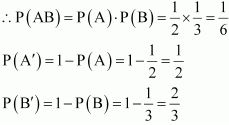i. Probability that the problem is solved = P(A ∪ B)
= P(A) + P(B) - P(AB)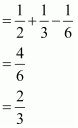(ii) Probability that exactly one of them solves the problem is given by P(A). P(B') + P(B).P(A')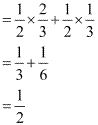15. One card is drawn at random from a well-shuffled deck of 52 cards. In which of the following case is the events E and F independent?
(i) E : ‘the card drawn is a spade’
F : ‘the card drawn is an ace’
(ii) E: ‘the card drawn is black’
F: ‘the card drawn is a king’
(iii) E: ‘the card drawn is a king or queen’
F: ‘the card drawn is a queen or jack’

Solution
(i) In a deck of  52 cards, 13 cards are spades and 4 cards are aces.
∴ P(E) = P(the card drawn is a spade) = 13/52 = 1/4
∴ P(F) = P(the card drawn is an ace) = 4/52 = 1/13
In the deck of cards, only 1 card is an ace of spades.
P(EF) = P(the card drawn is spade and an ace) = 1/52
P(E) × P(F) = 1/4 ×  1/13 = 1/52 = P(EF)
⇒ P(E) × P(F) = P(EF)
Therefore, the events E and F are independent.

(ii) In a deck of 52 cards, 26 cards are black and 4 cards are kings.
∴ P(E) = P(the card drawn is black) = 26/52 = 1/2
∴ P(F) = P(the card drawn is a king) = 4/52 = 1/13
In the pack of 52 cards, 2 cards are black as well as kings.
∴ P (EF) = P(the card drawn is a black king) = 2/52 = 1/26
P(E) × P(F) = 1/2 × 1/13 = 1/26 = P(EF)
Therefore, the given events E and F are independent.

(iii) In a deck of 52 cards, 4 cards are kings, 4 cards are queens, and 4 cards are jacks.
∴ P(E) = P(the card drawn is a king or a queen) = 8/52 = 2/13
∴ P(F) = P(the card drawn is a queen or a jack) = 8/52 = 2/13
There are 4 cards which are king or queen and queen or jack.
∴ P(EF) = P(the card drawn is a king or a queen, or queen or a jack)
= 4/52 = 1/13
P(E) × P(F) = 2/13. 2/13 = 4/169 ≠ 1/13
⇒ P(E). P(F) ≠ P(EF)
Therefore, the given events E and F are not independent.

16. In a hostel, 60% of the students read Hindi newspaper, 40% read English newspaper and 20% read both Hindi and English news papers. A student is selected at random.
(a) Find the probability that she reads neither Hindi nor English news papers.
(b) If she reads Hindi news paper, find the probability that she reads English news paper.
(c) If she reads English news paper, find the probability that she reads Hindi news paper.
Solution
Let H denote the students who read Hindi newspaper and E denote the students who read English newspaper.
It is given that,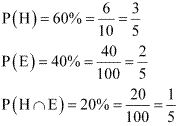(i) Probability that a student reads Hindi or English newspaper is,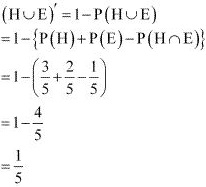(ii) Probability that a randomly chosen student reads English newspaper, if she reads Hindi news paper, is given by P (E|H).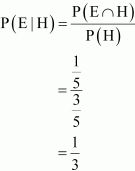(iii) Probability that a randomly chosen student reads Hindi newspaper, if she reads English newspaper, is given by P (H|E).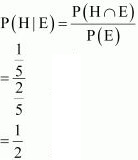17. The probability of obtaining an even prime number on each die, when a pair of dice is rolled is
(A) 0
(B) 1/3
(C) 1/12
(D) 1/36

Solution

When two dice are rolled, the number of outcomes is 36.
The only even prime number is 2.
Let E be the event of getting an even prime number on each die.
∴ E = {(2, 2)}
⇒ P(E) = 1/36
Therefore, the correct answer is D.

18. Two events A and B will be independent, if
(A) A and B are mutually exclusive
(B) P(A'B') = [1 - P(A)][1-P(B)]
(C) P(A) = P(B)
(D) P(A) + P(B) = 1

Solution

Two events A and B are said to be independent, if p(AB) = P(A) × P(B)
Consider the result given in alternative B.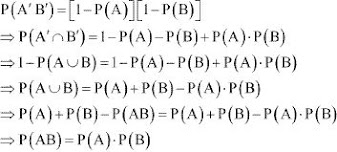This implies that A and B are independent, if P(A'B') = [1 - P(A)] [1 - P(B)]
Distracter Rationale
A. Let P(A) = m, P(B) = n, 0 < m, n < 1
A and B are mutually exclusive.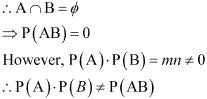C. Let A : Event of getting an odd number on throw of a die = {1, 3, 5}
⇒ P(A) = 3/6 = 1/2
B:  Event of getting an even number on throw of a die = { 2, 4, 6}
P(B) = 3/6 = 1/2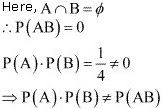D. From the above example, it can be seen that,
P(A) + P(B) = 1/2 + 1/2 = 1
However, it cannot be inferred that A and B are independent.
Thus, the correct answer is B.